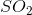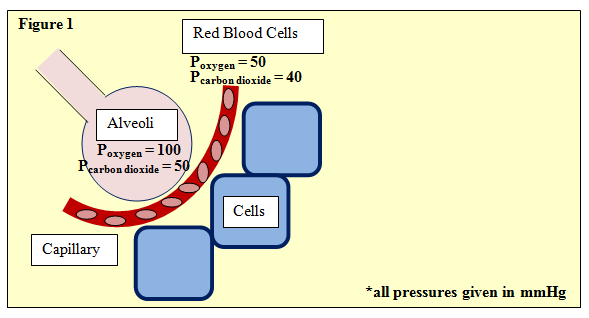# MCAT Physical : Lewis Dot Structures

## Example Questions

### Example Question #1 : Lewis Dot Structurescan be represented by three different Lewis diagrams. What is best term for this phenomenon?

Epimers

Isotopes

Resonance structures

Isomers

Resonance structures

Explanation:

The answer is resonance structures. When different Lewis structures can be drawn for a single molecule, the molecule exists as a composite of these structures, which are called resonance structures.

Isotopes refers to multiple nuclear compositions for a single element, based on varying numbers of neutrons. Isomers are different configurations of a given molecular formula, based on geometry and orientation. Epimers are a specific class of isomers involving a single stereocenter.

### Example Question #1 : Lewis Dot Structures

Diffusion can be defined as the net transfer of molecules down a gradient of differing concentrations. This is a passive and spontaneous process and relies on the random movement of molecules and Brownian motion. Diffusion is an important biological process, especially in the respiratory system where oxygen diffuses from alveoli, the basic unit of lung mechanics, to red blood cells in the capillaries.Figure 1 depicts this process, showing an alveoli separated from neighboring cells by a capillary with red blood cells. The partial pressures of oxygen and carbon dioxide are given. One such equation used in determining gas exchange is Fick's law, given by:

ΔV = (Area/Thickness) · Dgas · (P1 – P2)

Where ΔV is flow rate and area and thickness refer to the permeable membrane through which the gas passes, in this case, the wall of the avlveoli. P1 and P2 refer to the partial pressures upstream and downstream, respectively. Further, Dgas­, the diffusion constant of the gas, is defined as:

Dgas = Solubility / (Molecular Weight)^(1/2)

How many total valence electrons does carbon dioxide contain?

10

22

16

24

16

Explanation:

This is a straightforward question that has little to do with the passage, but it's a concept almost certainly to be seen on the MCAT.

Make sure to understand the concept of valence electrons and what that number may tell you about bonding properties. Questions like these are essentially "freebies," and should be answered without hesitation.

### Example Question #3 : Lewis Dot Structures

How many valence electrons do boron and nitrogen have?

Boron has five valence electrons.

Nitrogen has three valence electrons.

Boron has three valence electrons.

Nitrogen has five valence electrons.

Boron has three valence electrons.

Nitrogen has four valence electrons.

Boron has seven valence electrons.

Nitrogen has eight valence electrons.

Boron has three valence electrons.

Nitrogen has five valence electrons.

Explanation:

To determine the number of electrons an atom has, you must look at which column the atom is in on the periodic table. Boron is in column 3A, so it has three valence electrons. Nitrogen is in column 5A, so it has five valence electrons.

You should be familiar with common elements, like nitrogen, without looking at the periodic table. This will save you time on the exam.

### All MCAT Physical Resources